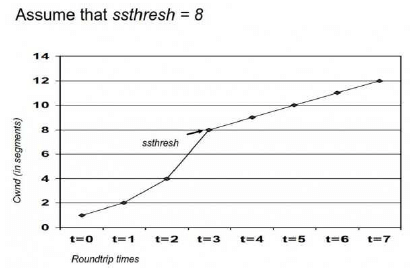# Test: Communication & Congestion Control

## 10 Questions MCQ Test RRB JE for Computer Science Engineering | Test: Communication & Congestion Control

Description
Attempt Test: Communication & Congestion Control | 10 questions in 30 minutes | Mock test for Computer Science Engineering (CSE) preparation | Free important questions MCQ to study RRB JE for Computer Science Engineering for Computer Science Engineering (CSE) Exam | Download free PDF with solutions
QUESTION: 1

### Assume that each character code consists of 8 bits. The number of characters that can be transmitted per second through anasynchronous serial line at 2400 baud rate, and with two stop bits is

Solution:

Total bit per character = 8 bit data + 2 stop bit +1 start bit (#) = 11 bits
no of characters = 2400/11 = 218.18
Since it is asked for transmitted characters we take floor and answer is 218.

QUESTION: 2

### Purpose of a start bit in RS 232 serial communication protocol is

Solution:

A) Because RS 232 requires a start before each byte transmission for synchronization..

QUESTION: 3

### In serial data transmission, every byte of data is padded with a '0' in the beginning and one or two '1's at the end of bytebecause

Solution:

In serial communication in beginning '0' is padded as start bit and one or two '1's are padded as stop bit.
and those bits are for synchronize receiver

QUESTION: 4

How many 8-bit characters can be transmitted per second over a 9600 baud serial communication link using asynchronousmode of transmission with one start bit, eight data bits, two stop bits and one parity bit?

Solution:

The baud rate is the rate at which information is transferred in a communication channel. Serial ports use two-level (binary) signaling, so the data rate in bits per second is equal to the symbol rate in bauds.

"9600 baud" means that the serial port is capable of transferring a maximum of 9600 bits per second."
So, transmission rate here = 9600 bps
An eight bit data (which is a char) requires 1 start bit, 2 stop bits and 1 parity bit = 12 bits.
So, number of characters transmitted per second = 9600 / 12 = 800

QUESTION: 5

A serial transmission T1 uses 8 information bits, 2 start bits, 1 stop bit and 1 parity bit for each character. A synchronoustransmission T2 uses 3 eight-bit sync characters followed by 30 eight-bit information characters. If the bit rate is 1200bits/second in both cases, what are the transfer rates of T1 and T2?

Solution:

T1: 1 char. = ( 8 + 2 + 1 + 1) = 12 bit
Transfer Rate = 1200/12 = 100 char/sec.
T2: Transfer character in bits = 24 + 240 = 264 bits
In 264 = 30 character
Then 1200 = ?
264/30 = 1200/X
X = 136.3 character / sec.
so correct option is (C)

QUESTION: 6

Let us consider a statistical time division multiplexing of packets. The number of sources is 10. In a time unit, a source transmits a packet of 1000 bits. The number of sources sending data for the first 20 time units is 6, 9, 3, 7, 2, 2, 2, 3, 4, 6,1, 10, 7, 5, 8, 3, 6, 2, 9, 5 respectively. The output capacity of multiplexer is 5000 bits per time unit. Then the average number of back logged of packets per time unit during the given period is

Solution:

Here we can spent at max 5 packets per Time unit 5000 /1000.
So Whatever which is not sent is backlog.
So First Time Unit => 6 ,
Second time unit => 9 + 1 (One from Previous Time Unit)
Total Backlog this way = 1+5+3+5+2+0+0+0+0+1+0+5+7+7+10+8+9+6+10+10=89
Avg Backlog=89/20=4.45
The average number of backlogged of packets per time unit during the given period is 4.45 , (Option B) .

QUESTION: 7

On a TCP connection, current congestion window size is Congestion Window = 4 KB. The window size advertised by the receiver is Advertise Window = 6 KB. The last byte sent by the sender is Last Byte Sent = 10240 and the last byte acknowledged by the receiver is Last Byte Acked = 8192. The current window size at the sender is

Solution:

Ans should be (B)
Current Sender window = min (Congestion Window, Advertised Window)= min(4KB, 6KB)= 4KB

QUESTION: 8

In the slow start phase of the TCP congestion algorithm, the size of the congestion window

Solution:increase is exponential in the Slow Start Phase.

QUESTION: 9

Consider an instance of TCP’s Additive Increase Multiplicative Decrease (AIMD) algorithm where the window size at the startof the slow start phase is 2 MSS and the threshold at the start of the first transmission is 8 MSS. Assume that a time out occurs during the fifth transmission. Find the congestion window size at the end of the tenth transmission.

Solution:

The Answer is correct , but method of solving is wrong .
At
t=1, =>2mss
t=2, =>4mss
t=3, =>8mss
t=4, =>9mss (after threshold additive increase)
t=5, =>10mss (fails)
Threshold will be reduced by n/2 i.e. 10/2 = 5.
t=6, =>2mss
t=7 =>4mss
t=8, =>5mss
t=9, =>6mss
t=10, =>7mss.
So at the end of 10th transmission congestion window size will be 8 mss.

QUESTION: 10

Let the size of congestion window of a TCP connection be 32 KB when a timeout occurs. The round trip time of the connection is 100 msec and the maximum segment size used is 2 KB. The time taken (in msec) by the TCP connection to get back to 32 KB congestion window is _________.

Solution:Use Code STAYHOME200 and get INR 200 additional OFF Use Coupon Code